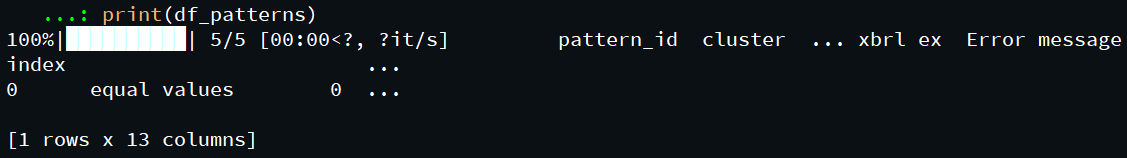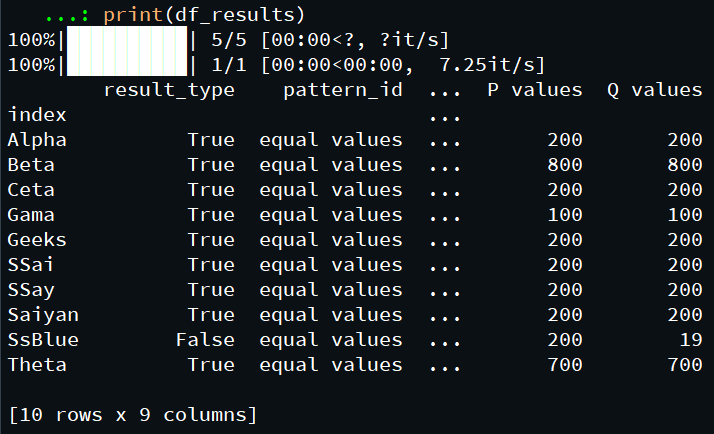Skip to content
Related Articles
Identifying patterns in DataFrames using Data-Pattern Module
• Last Updated : 24 Oct, 2020

Prerequisites: Pandas Module, Pandas Data frame

Pandas is an open-source library that is built on top of NumPy library. It is a Python package that offers various data structures and operations for manipulating numerical data and time series. It is mainly popular for importing and analyzing data much easier. Pandas are fast and it has high-performance & productivity for users.

Data Frame is two-dimensional size-mutable, potentially heterogeneous tabular data structure with labeled axes (rows and columns) in Pandas. A Data frame is a two-dimensional data structure, i.e., data is aligned in a tabular fashion in rows and columns. Pandas data frame consists of three principal components, the data, rows, and columns.

Data Pattern module, In order to find the simple data patterns in the data frame we will use the data-patterns module in python, this module is used for generating and evaluating patterns in structured datasets and exporting to Excel and JSON and transforming generated patterns into Pandas code.

### Installation:

```pip install data-patterns
```

### Step-by-step Approach:

Import required modules.

Assign data frame.

Create pattern-mixer object with the data frame as a constructor argument.

Call find() method of the pattern-mixer object to identify various patterns in the data frame.

### Implementation:

Below are some programs based on the above approach:

## Python3

 `# importing the data_patterns module``import` `data_patterns`` ` ` ` `# importing the pandas module``import` `pandas as pd`` ` ` ` `# creating a pandas dataframe``df ``=` `pd.DataFrame(columns``=``[``'Name'``, ``'Grade'``, ``'value1'``,``                           ``'Value2'``, ``'Value3'``, ``'Value4'``, ``'value5'``],``                  ``data``=``[[``'Alpha'``, ``'A'``, ``1000``, ``800``, ``0``, ``200``, ``200``],``                        ``[``'Beta'``, ``'B'``, ``4000``, ``0``, ``3200``, ``800``, ``800``],``                        ``[``'Gama'``, ``'A'``, ``800``, ``0``, ``700``, ``100``, ``100``],``                        ``[``'Theta'``, ``'B'``, ``2500``, ``1800``, ``0``, ``700``, ``700``],``                        ``[``'Ceta'``, ``'C'``, ``2100``, ``0``, ``2200``, ``200``, ``200``],``                        ``[``'Saiyan'``, ``'C'``, ``9000``, ``8800``, ``0``, ``200``, ``200``],``                        ``[``'SSai'``, ``'A'``, ``9000``, ``0``, ``8800``, ``200``, ``200``],``                        ``[``'SSay'``, ``'A'``, ``9000``, ``8800``, ``0``, ``200``, ``200``],``                        ``[``'Geeks'``, ``'A'``, ``9000``, ``0``, ``8800``, ``200``, ``200``],``                        ``[``'SsBlue'``, ``'B'``, ``9000``, ``0``, ``8800``, ``200``, ``19``]])`` ` ` ` `# setting datag=frame index``df.set_index(``'Name'``, inplace``=``True``)`` ` ` ` `# creating a pattern mixer object``miner ``=` `data_patterns.PatternMiner(df)`` ` ` ` `# finding the pattern in the datframe``# name is optional``# other patterns which can be used  ‘>’, ‘<’, ‘<=’, ‘>=’, ‘!=’, ‘sum’``df_patterns ``=` `miner.find({``'name'``: ``'equal values'``,``                          ``'pattern'``: ``'='``,``                          ``'parameters'``: {``"min_confidence"``: ``0.5``,``                                         ``"min_support"``: ``2``,``                                         ``"decimal"``: ``8``}})`` ` ` ` `# priniting the dataframe pattern``print``(df_patterns)`

Output:The data items value4 and value5 are having equal patterns with support of 9 and 1 exception.
Also, this data can be analyzed in proper format with the help of analyze() method, below is the improved program:

## Python3

 `# importing the data_patterns module``import` `data_patterns`` ` ` ` `# importing the pandas module``import` `pandas as pd`` ` ` ` `# creating a pandas dataframe``df ``=` `pd.DataFrame(columns``=``[``'Name'``, ``'Grade'``, ``'value1'``,``                           ``'Value2'``, ``'Value3'``, ``'Value4'``, ``'value5'``],``                  ``data``=``[[``'Alpha'``, ``'A'``, ``1000``, ``800``, ``0``, ``200``, ``200``],``                        ``[``'Beta'``, ``'B'``, ``4000``, ``0``, ``3200``, ``800``, ``800``],``                        ``[``'Gama'``, ``'A'``, ``800``, ``0``, ``700``, ``100``, ``100``],``                        ``[``'Theta'``, ``'B'``, ``2500``, ``1800``, ``0``, ``700``, ``700``],``                        ``[``'Ceta'``, ``'C'``, ``2100``, ``0``, ``2200``, ``200``, ``200``],``                        ``[``'Saiyan'``, ``'C'``, ``9000``, ``8800``, ``0``, ``200``, ``200``],``                        ``[``'SSai'``, ``'A'``, ``9000``, ``0``, ``8800``, ``200``, ``200``],``                        ``[``'SSay'``, ``'A'``, ``9000``, ``8800``, ``0``, ``200``, ``200``],``                        ``[``'Geeks'``, ``'A'``, ``9000``, ``0``, ``8800``, ``200``, ``200``],``                        ``[``'SsBlue'``, ``'B'``, ``9000``, ``0``, ``8800``, ``200``, ``19``]])`` ` `# setting datag=frame index``df.set_index(``'Name'``, inplace``=``True``)`` ` ` ` `# creating a pattern mixer object``miner ``=` `data_patterns.PatternMiner(df)`` ` ` ` `# finding the pattern in the datframe``# name is optional``# other patterns which can be used  ‘>’, ‘<’, ‘<=’, ‘>=’, ‘!=’, ‘sum’``df_patterns ``=` `miner.find({``'name'``: ``'equal values'``,``                          ``'pattern'``: ``'='``,``                          ``'parameters'``: {``"min_confidence"``: ``0.5``,``                                         ``"min_support"``: ``2``,``                                         ``"decimal"``: ``8``}})`` ` ` ` `# getting the analyized dataframe``df_results ``=` `miner.analyze(df)`` ` ` ` `# prinitng the analyized results``print``(df_results)`

Output:As we can see here, various patterns are identified between different data items present in the data frame.

Attention geek! Strengthen your foundations with the Python Programming Foundation Course and learn the basics.

To begin with, your interview preparations Enhance your Data Structures concepts with the Python DS Course. And to begin with your Machine Learning Journey, join the Machine Learning – Basic Level Course

My Personal Notes arrow_drop_up